# Loads and Forces Acting on Retaining Wall and Their Calculations [PDF]Reading time: 1 minute

Various types of loads and forces act on a retaining wall, and their calculation is essential for its design. These forces on the retaining wall depends on multiple factors which are discussed.

## Loads and Forces Acting on Retaining Wall

There are various types of loads and forces acting on retaining wall, which are:

1. Lateral earth pressure
4. Wind on projecting stem
5. Impact forces
6. Seismic earth pressure
7. Seismic wall self-weight forces

Retaining wall design could include any or all of loads and forces which are explained in the following sections:

### 1. Lateral Earth Pressure Acting on Retaining Wall

The main purpose of retaining wall construction is to retain soil; that is why soil lateral earth pressure is a major concern in the design. Sliding soil wedge theory is the basis for most of the theories by which lateral earth pressure is computed.

The wedge theory suggests that a triangular wedge of soil would slide down if the retaining wall were removed suddenly, and the wall has to sustain this wedge soil. Figure 1 shows free body lateral forces acting on retaining walls.Fig 1: Free body of lateral forces acting on retaining wall

Coulomb and Rankine equations are two major formulas which are used to compute lateral earth pressure:

#### The Coulomb method of Lateral Earth Pressure Calculation

This equation takes backfill slope, friction angle at wall face, rupture plan angle, and internal friction angle into consideration:

Where:
Ka: Coefficient of active pressure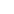: Angle of internal friction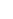: Angle of backfill slope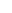: Angle of friction between soil and wall (2/3to 1/2is assumed)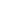: Slope angle of the wall which is measured from horizontal (equal to 90 degrees for the vertical wall)

Furthermore, in the case of flat level backfill soil, considering zero friction at soil-wall interface, and soil-sidewall is vertical, the coulomb equation is reduced to the following:

#### The Rankine method of Lateral Earth Pressure Calculation

This equation, which derived by William Rankine, is the development of the coulomb formula. The Rankine method does not take the friction between the wall and soil into account.

This makes it a conservative way of designing retaining walls. The Rankine lateral earth pressure equation is the same for both zero-wall friction and level backfill soil:

Where:: Backfill slope angle: Internal friction angle of soil

Rankine equation is rearranged when backfill is level as:

### 2. Surcharge loads Acting on Retaining Wall

Surcharge loads acting on retaining walls are additional vertical loads that used to the backfill soil above the top of the wall. It can be either dead loads, for example, sloping backfill above the wall height or live load, which could result from the highway or parking lot, paving, or adjacent footing.

Live load surcharge is considered when vehicular actions act on the surface of backfill soil at a distance, which equal or less than the wall height from the wall back face. Active pressure from uniform surcharge is explained in Figure 2.Fig 2: Active pressure from a uniform surcharge against the retaining wall

Where: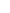: is the density of soil
W: is the uniform surcharge load
H: is the height of the wall

P1=Ka WH  --> Equation 7

P2=0.5KaH2   -->Equation 8

#### There are various types of surcharge loads such as:

1. Highway surcharges
2. Backfill compaction surcharge

### 3. Axial Forces Acting on Retaining Wall

Overturning resistance on the retaining wall is provided by axial loads. Different types of axial load will be discussed in the following sections:

#### a) Vertical loads on the stem

These loads might be resulted from beam reactions, bridge, or lodger and applied to the stem directly.

For most critical conditions, it is not necessary to consider live load from dead load separately because the axial live load on the stem increases is resisting moments and soil bearing pressure.

Point vertical loads on walls are considered to be spread downward in a slope of two vertical to one horizontal. Consequently, there will be rather low compressive stresses at the base of the wall; girder reactions on walls is an example of vertical point load.

Moreover, bearing stresses that directly under girder or beams reactions must be checked in addition to take eccentricity into account with respect to the stem centerline since it influences the stability and design of the stem.

Finally, it is worth mentioning that un-conservative results might be produced by acting live loads at negative eccentricity toward backfill.

#### b) Soil weight

It is the weight of the soil above toe and heel of the retaining wall.

#### c) Structural weight

It includes the weight of the footing and stem, which added to the bearing pressure of the soil and help stability against sliding and overturning.

#### d) Vertical component of active pressure

It is another vertical load; the resultant earth pressure action line is at an angle from horizontal provided that backfill soil is sloped.

The angle is equal to the backfill slope angle according to Rankine formula and is the same as the soil-stem friction angle according to the coulomb formula. This inclined active pressure has two components that include horizontal and vertical.

The latter is employed as added sliding resistance, decrease soil pressure, and increases withstand against overturning.

### 4. Wind Forces on Projecting Stem

Wind pressure generates an overturning force when retaining wall is exposed and extends above grade. A common formula used to compute wind pressure is as follow:

F=0.0026V2  --> Equation 9

Where:
F: wind pressure
V: Velocity of the wind

According to ASCE 7 design wind pressure (F) is calculated using the following simplified formula:

F=qz GGf    --> Equation 10

Where:
G: is the gust factor (0.85 can be used)
Gf: Commonly taken as 1.2
qz: is the velocity pressure at mid height and can be calculated using the following formula:

qz=0.613Kz Kzt Kd V2  --> Equation 11

Where:
Kz: wind directionality factor, can be determined in section 26.6 of ASCE 7-10
Kzt: Velocity pressure exposure coefficient, can be determined section 26.6 of ASCE 7-10
Kd: Topographic factor see section, can be determined 26.6 of ASCE 7-10
V: Basic wind speed in m/s

### 5. Impact loads Acting on Retaining Wall

Design retaining wall for car bumper might be necessary when the wall extends above grade, and the parking area is close to it. When retaining wall is designed for impact loads, the stem should be checked at equally spaced points along stem length from top to the bottom as impact load spread at the greater length of the stem. Moreover, use the slope of two vertical to one horizontal for spreading impact load.

Forces related to earthquake is covered in seismic design of retaining wall.

1. Which are the various types of loads and forces acting on retaining wall?

The various types of loads and forces acting on retaining wall are-
1. Lateral earth pressure
4. Wind on projecting stem
5. Impact forces
6. Seismic earth pressure
7. Seismic wall self-weight forces

2. What are the various types of surcharge loads acting on a retaining wall?

The various types of surcharge loads acting on a retaining wall are-
1. Highway surcharges
2. Backfill compaction surcharge

3. What is the equation for the Coulomb method of Lateral Earth Pressure?

The equation for Coulomb method of Lateral Earth Pressure is given asWhere:
KaCoefficient of active pressure: Angle of internal friction: Angle of backfill slope: Angle of friction between soil and wall (2/3to 1/2is assumed): Slope angle of the wall which is measured from horizontal (equal to 90 degrees for the vertical wall)

4. What is the equation for the Rankine method of Lateral Earth Pressure?

The equation for the Rankine method of Lateral Earth Pressure is given as-Where:: Backfill slope angle: Internal friction angle of soil